# KSEEB Solutions for Class 6 Maths Chapter 7 Fractions Ex 7.3

Students can Download Chapter 7 Fractions Ex 7.3 Questions and Answers, Notes Pdf, KSEEB Solutions for Class 6 Maths helps you to revise the complete Karnataka State Board Syllabus and score more marks in your examinations.

## Karnataka State Syllabus Class 6 Maths Chapter 7 Fractions Ex 7.3

Question 1.
Write the fractions. Are all the these fractions equivalent?Solution:
a) In the given circles, 1 out of 2, 2 out of 4, 3 out of 6, and 4 out of 8 equal part of shaded respectively. Therefore these circles represent.
Also, all these fractions are equivalent.
b) In the given rectangles, 4 out of 12, 3 out of 9, 2 out of 6, 1 out of 3, and 6 out of 15 equal parts (i.e, circles) are shaded respectively Therefore these rectangles represent, Not, not all of these fractions are equivalent.

Question 2.
Write the fractions and pair up the equivalent fractions from each row.Solution:
a) Here, 1 parts is shaded out of 2 equal parts ( i,e., rectangle) Hence this, Figure represents a fraction $$\frac{1}{2}$$
b) Here, 4 parts is shaded out of 6 equal parts ( i,e., rectangle) Hence this, Figure represents a fraction $$\frac{4}{6}$$ = $$\frac{2}{3}$$
c) Here, 3 parts is shaded out of9 equal parts ( i,e., rectangle) Hence this, Figure represents a fraction $$\frac{3}{9}$$ = $$\frac{1}{3}$$
d) Here, 2 parts is shaded out of 8 equal parts ( i,e., rectangle) Hence this, Figure represents a fraction $$\frac{2}{8}$$ = $$\frac{1}{4}$$
e) Here,3 parts is shaded out of4 equal parts ( i,e., rectangle) Hence this, Figure represents a fraction $$\frac{3}{4}$$
i) Here,6 parts are shaded out of 18 equal parts (i.e, triangles) Hence, this figure represents a fraction $$\frac{6}{18}$$ = $$\frac{1}{3}$$
ii) Here, 4 parts are shaded out of 8 equal parts (i,e, rectangle) Hence, this figure represents a fraction $$\frac{4}{8}$$ = $$\frac{1}{2}$$
iii) Here, 12 parts are shaded out o f 16 equal parts (i.e , squares) Hence, this figure $$\frac{12}{16}$$ = $$\frac{3}{6}$$
iv) Here, 8 parts are shaded out of 12 equal parts (i,e . rectangle) Hence, this figure represents a fraction $$\frac{8}{12}$$ = $$\frac{2}{3}$$
v) Here,4 parts are shaded out of 16equal parts (i,e , triangles) Hence, this figure represents a fraction $$\frac{4}{16}$$ = $$\frac{1}{4}$$
Now these figures can be, matched correctly as (a)(ii), (b)(iv), (c)(i), (d)(v), (e)(iii).Question 3.
Replace and mnsq2 in each of the following by the correct number: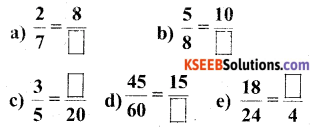Solution:Hence, & mnsq2 Can be replaced by 28.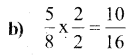Hence, & mnsq2 Can be replaced by 16.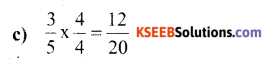Hence, & mnsq2 Can be replaced by 12.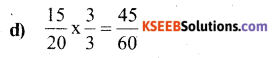Hence, & mnsq2 Can be replaced by 20.Hence, & mnsq2 Can be replaced by 3.

Question 4.
Find the equivalent fraction of $$\frac{3}{5}$$ having
a) denominator 20
b) numerator 9
c) denominator 30
d) numerator 27
Solution:
a)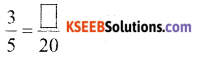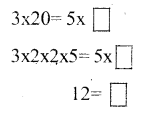Hence, the required fraction is $$\frac{12}{20}$$b)Hence, the required fraction is $$\frac{18}{30}$$

d)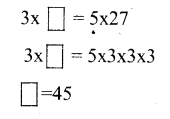Hence, the required fraction is $$\frac{27}{45}$$

Question 5.
Find the equivalent fraction of $$\frac{36}{48}$$ with
a) numerator 9
b) denominator 4
Solution:
a)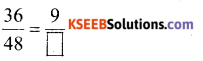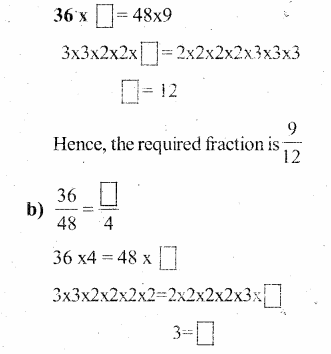Hence, the required fraction is $$\frac{3}{4}$$

Question 6.
Check whether the given fractions are equivalent:Solution:
a)Clearly, both the fractions are equivalent.

b)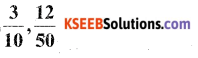Clearly, both the fractions are not equivalent.

c)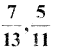clearly, both the fractions are not equivalentQuestion 7.
Reduce the following fractions to simplest form:Solution: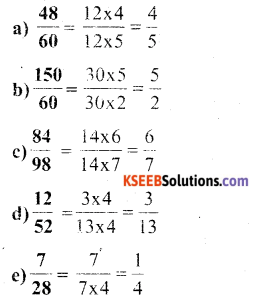Question 8.
Ramesh had 20 pencils, Sheelu had 50 pencils and Jamaal had 80 pencils. After 4 months, Ramesh used tip 10 pencils, Sheelu used up 25 pencils and Jamaal used up 40 pencils. What fraction did each use up? Check if each has used up an equal fraction of her/ his pencils?
Solution:
Fraction used by Ramesh = $$\frac{10}{20}=\frac{1}{2}$$
Fraction used by sheelu = $$\frac{25}{50}=\frac{1}{2}$$
Fraction used by Jamaal = $$\frac{40}{80}=\frac{1}{2}$$
Yes, all of them used equal fraction of pencils, i.e., $$\frac{1}{2}$$Question 9.
Match the equivalent fractions and write two more for each.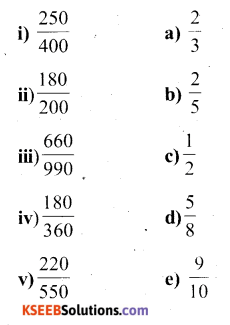Solution:
i)Two more fractions are $$\frac{25}{40}, \frac{30}{48}$$

ii)Two more fractions are $$\frac{18}{20}, \frac{27}{30}$$

iii)Two more fractions are $$\frac{20}{30}, \frac{200}{300}$$

iv)Two more fractions are $$\frac{20}{40}, \frac{30}{60}$$v)Two more fractions are $$\frac{20}{40}, \frac{40}{100}$$
Now these can be matched as
(i) – (d),
(ii) – (e),
(iii) – (a),
(iv) – (c),
(v) – (b)

error: Content is protected !!